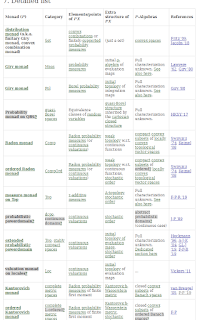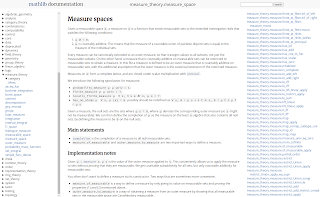### Domain types based on probability monads

(Important:  I did not create the image shown in blogger preview - it is from NCat Lab website!)

## Composed stochastic proof for low-dimensional applications

Following up on previous post on Composed Stochastic Proof, we now enumerate:

### What the typed world owes the authored world

Compile-time access to categorical knowledge about probability monads (overview page at NCat Lab site) over our dependent type system for measure theory (at MathLib/Lean site).

To knit this knowledge into useful, portable applications, we proceed with four major tasks:

Task 1) Starting from a shared library conceptual math knowledge, we identify applicable categories that are useful in our application model.  The NCatLab website is a great current resource on existing work.  The page on monads of probability, measures, and valuations contains this summary table of the most useful, widely known functional probability concepts. These are the core ingredient math concepts needed for robust, general processing of probability models in functional computing environments.Probability monads overview at NCat Lab

Task 2) Build upon our chosen categories some verified theories of useful domain types, by  wiring together desirable concepts from a proof library such as MathLib (formal math library developed by Lean prover community).

These domain types formally define our required categories and functions.  This definition process leverages the ideas of dependent type theory, which we have delved into in previous posts here.  For app developers, what matters is that the MathLib package for measure_theory provides reusable knowledge in the form of a set of types (propositions) and proofs.  These mathematical types are the  foundation of interoperability of our powerful math concepts (from Task #1 above), which are primarily categories and functions.

Any strongly-typed programming language may define corollary types with equivalent meaning and (provably) equivalent data serializations.  These two forms of equivalence correspond to intensional and extensional equality, which may each be reasoned about interactively among humans and machines.MathLib docs for measure theory types + proofs

Having adopted such a formalism, then applying our typed concepts reduces to component assembly:

Task 3) Assemble a compliant, sufficient network of components to verifiably implement the stated type contracts.  The stronger and more faithful the types are to our proof model #2, the more productive this assembly effort #3.

Choosing and configuring or building this type-compliant execution environment is a primary cost we seek to reduce, by making the required infrastructure universally available in advanced strong-typed environments such as F*, Idris, Agda as well as modern hybrids like Scala 3, Rust, and Julia.   We also seek general compatibility with existing environments for probabilistic modeling including distribution semantics, auto differentiation, algebraic and symbolic representations such as Stan, TensorFlow, Ranier, Keanu, λPSI, Hakaru, PRISM, OpenMath, and well known open scientific computing platforms like R, Python, Octave, SciLab.

Goals:

Axiomagic is strongly available in dependent-typed environments with proof support.

Axiomagic is available in strong-typed environments.

Axiomagic is compatible with dynamically-typed environments.

With these choices made, we obtain a compiled, deployable space of domain types.
What remains is data authoring, generation, and/or ingest, as appropriate for the application.

Task 4) Supply a compliant, sufficient set of instance data (authored, generated and/or ingested) to
accomplish useful work.

This process is broadly familiar to most practitioners.  What our method adds is the well defined target contract of the domain types constructed through Tasks #1,2,3.  This contract allows for verifiable data streams with precisely defined semantics, facilitating high quality outcomes for applications.

### Performance and Correctness of Strict and Relaxed implementations

As we apply methods ensuring formal correctness of our results, naturally performance concerns will be raised.  In general we expect that attaching strong guarantees may often inhibit scalability of computation in well known ways.   We apply the common principle that guarantees are most needed in interactive environments for design and experimentation.  Production deployments will generally use only pre-approved constructs, with fewer explicit guarantees, while using the same immutable functions and semantic data structures defined in our interactive formal environments.  In the interactive environment, we commonly work with small dimensional systems, with high degrees of symbolic expression and element interconnection (such as correlation).

### Example Types, Components, and Data

Returning to our Asset Allocation example, we now work to represent useful general probability distributions for financial asset performance measures.  These distributions are defined using strongly typed monads, compatible with the MathLib types in the Measure_Theory package.  As our first attempt at component assembly, we build a Scala 2 implementation defining a programmer's domain type model (Task #3) and some data generators exercising the model (Task #4).  We expect to refine this domain type model for increasing levels of harmony with the MathLib measure_theory (from Task #2) and sufficient mathematical power from the Giry monad and related constructs (from Task #1).

We expect this same domain type model to eventually be strongly available (in Idris, Lean, F*, Agda, Coq), weakly available (in Stan, TensorFlow, Dotty, Julia, Rust, Typescript) and compatible (with Python, R, and everything else that can serialize data structures) as outlined previously.

First Event-Sample Space is any object X in the category FRCC_R_N
= Finite, rectilinear, convex, compact subsets of N-dimensional euclidean space over real numbers.

In informal terms, X is any finite N-box, and may be visualized and computed over as such.

Thus X is isomorphic to a cartesian product of compact closed intervals in R, each specified by an inclusive lower and upper bound:

•    ( [lower_1, upper_1] , [lower_2, upper_2] , ...  [lower_N, upper_N] )
• Where, for all n, lower_n <  upper_n
• Define width_n = upper_n - lower_n

Thus we obtain an obvious and immediate way to compute the euclidean (N-)volume of X.

• Vol_X = width_1 * width_2 *...*  width_N

X is a complete metric space equipped with the euclidean distance.
X is a compact Hausdorff topological space.
X is closed (as a subset of euclidean R^N), and includes its own boundary.
X is a Polish space.
The event space X is not itself treated as a vector space, nor is it equipped with any operators or special elements (other than its boundary elements).
In the special case where all the {width_n} are exactly 1, then X has volume 1 and is isomorphic to an N-cube, but here we do not make any further use of this special case.

Next we generate B_X = the Borel Space on X, which is a measurable space containing all open subsets of X.

Next we define an example of a measure: uniform_X as the uniform probability measure over B_X.("Uniform" means that uniform_X treats all events in X as equally probable).
Then we know that the continuous probability mass density of uniform_X is

• d_uniform_X =  1 / Vol_X

Similarly the probability of drawing an event in any measurable subset Y of X (where formally Y is an element of B_X) is just:

• Vol_Y / Vol_X
Note that Y need not be convex or even connected.  It may involve many small pieces of X, but (roughly speaking) each such piece must be open or compact.  The requirement that Y be a Borel subset of X ensures that Y is measurable. When we compute the (N-)volume of such a set, this is the same as the Lebesgue measure of Y.

Now let us step back from the technicalities and observe what we have defined.
We have specified a continuous finite event space which is natural to visualize and compute over,
with well defined distance and volume functions.  Unbiased uniform samples (from uniform_X) for this space may be generated as bounded N-vectors of real numbers. So we have a structure that straightforward to work with, both mentally and computationally.  However, we have not yet defined any interesting probability structures.

#### Projecting into lower dimensional spaces

X and B_X may be treated as the cartesian product of N (or fewer) separate topological spaces and associated Borel spaces.  We may informally say that X and B_X are "decomposed" or "projected" into these "smaller" component spaces.  These operations set the stage for the definition of marginal probability distributions.

#### Combining into higher dimensional spaces

The cartesian product of event spaces X1 and X2 (having Borel spaces B_X1, B_X2)
yields the joint event space
XX = (X1, X2), having Borel space B_XX.

If X1 has dimension N1 and X2 has dimension N2, then XX has dimension N1 + N2.

A distribution measure constructed over B_XX is a joint distribution over events in XX = (X1,X2).
Then integration may be used to project separate marginal distributions over B_X1 and B_X2
(and/or any of their component subspaces).

Now we define useful monadic structures for cleanly composable operations on objects like X, XX, B_X, B_XX, and uniform_X.

First let's introduce some more specific category theory and measure theory terminology, using links to some MathLib library constructs:

• X and XX (event spaces) are objects of category Top (Topological Spaces)
• B_X and B_XX (borel spaces) are objects of category Meas (Measurable Spaces)
• Borel is a functor which maps X to B_X, and maps XX to B_XX.
• uniform_X is an example of a probability measure, which is a function from B_X to [0,1], satisfying the constraint that the total measure of X is exactly 1.
• This constraint is important computationally, and quite interesting from a dependent typing perspective.
Another useful probability measure is δx, the Dirac Measure at x ∈ X, which assigns probability 1 to the single event x, transforming it into a deterministic outcome.  Thus we may construct any other probability measure on B_X as a weighted, normalized sum of δx measures.  Our monad structure is taking shape!

The uniform probability measure and the Dirac measure are both examples of Radon Measures.

Adapting the next two lines of text directly from the NCat Lab text on the Radon Measures page: